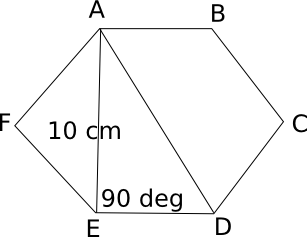# CAT Questions on Polygons With Solutions

0
2552

CAT Questions on Polygons With Solutions:

Geometry Polygons questions and answers for CAT. Polygons problems with solutions for CAT exam. Download excellent CAT preparation app to directly solve and practice questions.

Question 1: If the sum of interior angles of a polygon is 900 degrees, what is the number of diagonals it has?

a) 9
b) 14
c) 20
d) None of these

Question 2: In a regular pentagon PQRST, what is the ratio of the area of triangle PRS to the area of the pentagon PQRST?

a) 1 / (sin 72 + 4)
b) 1 / (cos 72 + 4)
c) 1 / (4 cos 72 + 1)
d) 1 / (4 sin 72 + 1)

Question 3: A regular polygon P has 135 diagonals. Find the exterior angle of the polygon P.

a) $$18^{\circ}$$
b) $$20^{\circ}$$
c) $$25^{\circ}$$
d) $$30^{\circ}$$

Question 4: A cylindrical pencil consists of a layer of wood surrounding a solid cylinder of graphite. The radius of the pencil is 6 mm, the radius of the graphite cylinder is 2 mm and the length of the pencil is 10 cm. Find the cost of the material used in a pencil, if the cost of wood is Rs. 0.70/cm3 and that of graphite is Rs. 2.80/cm3.

a) Rs. 7.85
b) Rs. 8.76
c) Rs. 9.36
d) Rs. 10.56

Question 5: If the shortest diagonal in a regular hexagon is 10 cm, what is the length of the longest diagonal (in cm)? Round your answer to the nearest integer. Given $$\sqrt3 = 1.732$$.

a) 12
b) 13
c) 14
d) 15

Solutions for CAT Questions on Polygons With Solutions:

Solutions:

The sum of interior angles for an ‘n’ sided polygon is (2n-4) \times 90 degrees.
If this value is 900, then the polygon is a heptagon.
The number of diagonals for an ‘n’ sided polygon = $$\frac{n(n-3)}{2}$$
= 14 for a heptagon.

Each angle of a regular pentagon is 108 degrees. In triangle PTS, angle T = 108 degrees, and PT = TS. So, angle TPS = angle TSP = 36 degrees.
Similarly, angle QPR = 36 degrees. So, angle SPR = 108 – (36 + 36) = 36 degrees
Also, PS = PR
Area of triangle PSR = ½ * PS * PR * sin 36
Area of the pentagon = Sum of areas of the three triangles = Area of triangle PTS + Area of triangle PSR + Area of triangle PQR
= ½ * PT * PS * sin (angle TPS) + ½ * PS * PR * sin 36 + ½ * PQ * PR * sin (angle QPR)
= ½ * PT * PS * sin 36 + ½ * PS * PR * sin 36 + ½ * PQ * PR * sin 36
Required ratio = ½ * PS * PR * sin 36 / (½ * PT * PS * sin 36 + ½ * PS * PR * sin 36 + ½ * PQ * PR * sin 36)
= PR / (PT + PR + PQ)
PT = PQ = SR
SR = 2 * PR cos (angle PSR) = 2 * PR * cos 72
Ratio = PR / (2 * PR cos 72 + PR + 2 * PR cos 72) = 1 / (4 cos 72 + 1)

Number of diagonals in a regular polygon = $$^nC_2 – n$$
=> $$^nC_2 – n$$ = 135
=> n = 18
Exterior angle = $$\frac{360}{n}$$ = $$\frac{360}{18}$$ = $$20^{\circ}$$

Volume of the whole pencil = $$3.6\pi$$ cm^3
Volume of graphite cylinder = $$0.4\pi$$ cm^3
Volume of wood part = $$3.6\pi-0.4\pi=3.2\pi$$ cm^3
Cost of the pencil
= $$3.2\times\frac{22}{7}\times0.7+0.4\times\frac{22}{7}\times2.8$$
= Rs. 10.56Sin 60 = AE/AD => AD = AE/sin 60 = $$10/(\sqrt3/2)$$ = $$20/\sqrt3$$ cm = 20/1.732 = 11.54 cm = 12 cm (approx.)